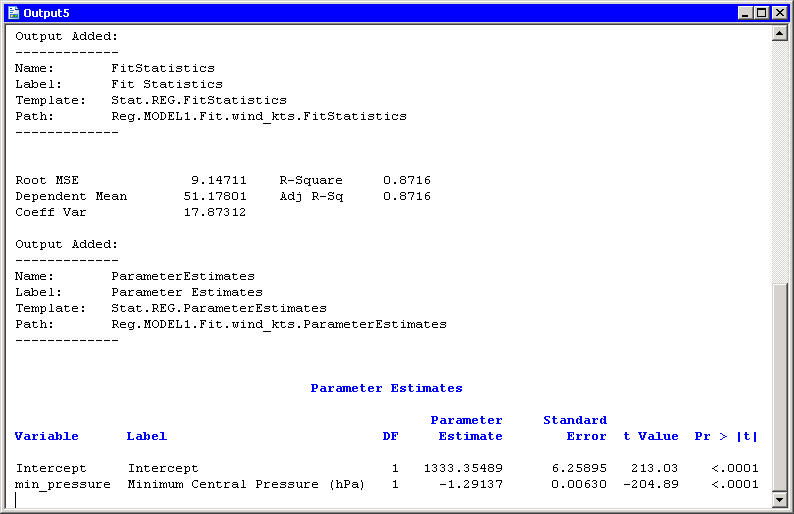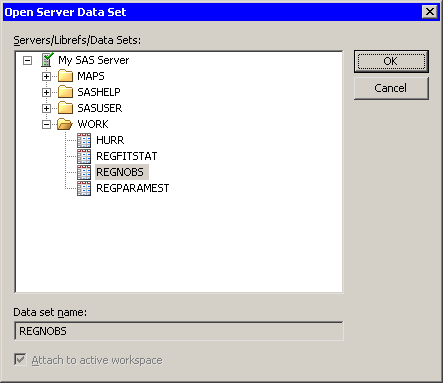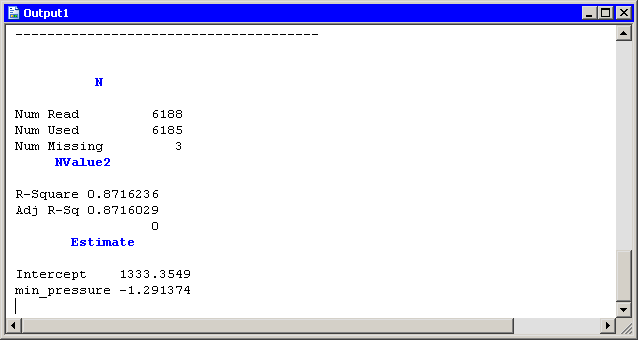Previous Page | Next Page

# Read Data from an ODS Table

The key to this chapter is knowing how to create SAS data sets directly from output tables by using the Output Delivery System (ODS). When you run a SAS procedure, the procedure typically creates one or more tables. By default, ODS sends the tables to the SAS listing, which in SAS/IML Studio is called the output window. But you can also tell ODS to send a table to a SAS data set. By subsequently reading the data set, you can read statistics from tables into your IMLPlus programs.

Suppose you perform a linear regression analysis with the REG procedure, and you want to read certain statistics into SAS/IML matrices. You might want to use the statistics in future computations, or you might want to use them in a title or legend for a plot.

To be specific, suppose you want to read the following statistics into your IMLPlus program: the number of observations used in the regression, the R-square value of the fitted model, and the slope and intercept of the least squares line.

Your first task is to determine the name of the ODS tables that contain the information you need. The "Details" section of the documentation for PROC REG includes a section about the names of ODS tables. You can also have ODS display the names by including the ODS TRACE ON statement before running your PROC REG statement.

This approach is illustrated in the following program. Type or copy the following statements into the program window, and select ProgramRun from the main menu.

```   declare DataObject dobj;
dobj = DataObject.CreateFromFile("Hurricanes");

dobj.WriteVarsToServerDataSet( {"wind_kts" "min_pressure"},
"Work", "Hurr", true );

submit;
ods trace on / listing;
proc reg data=Hurr;
model wind_kts = min_pressure;
run;
ods trace off;
endsubmit;
```

Figure 7.1 Printing ODS Tables NamesThe output is shown in Figure 7.1. The LISTING option in the ODS TRACE ON statement tells ODS to send the names of tables to the output window rather than to the SAS log. By scrolling through the output, you can see that the number of observations used in the regression is part of the "NObs" table. The R-square value of the fitted model is contained in the "FitStatistics" table. The slope and intercept of the least squares line are contained in the "ParameterEstimates" table. The following example uses the ODS OUTPUT statement to write these tables to data sets in Work.

Replace the SUBMIT/ENDSUBMIT block of statements in the program window with the following revised statements. Then select ProgramRun from the main menu.

```   submit;
proc reg data=Hurr;
model wind_kts = min_pressure;
ods output NObs = RegNObs
FitStatistics = RegFitStat
ParameterEstimates = RegParamEst;
run;
endsubmit;
```

These statements cause the REG procedure to create three new data sets in the Work library. You can examine each data set by opening it from the server into a data table as follows:

Select FileOpenServer Data Set from the main menu. The dialog box in Figure 7.2 appears.

• Expand the entry for your current SAS server.

• Expand the entry for the Work directory.

• Click the RegNObs data set.

• Click OK.

• Repeat the previous steps to open the RegFitStat and RegParamEst data sets.

• Figure 7.2 Opening a Server Data SetYou might notice that some data sets look different from the corresponding tables that are displayed in the output window. Some tables have nonprinting columns that are not visible in the output window but are written to the data set.

By opening each data set in turn, you can determine the name of the variable that contains the information that you want to read into SAS/IML matrices. The number of observations used in the regression is contained in the N variable in the RegNObs data set. The R-square value of the fitted model is contained in the NValue2 variable in the RegFitStat data set. The slope and intercept of the least squares line are contained in the Estimate variable of the RegParamEst data set.

The SAS/IML language provides statements to read and write server data to and from matrices. The USE statement opens a SAS data set, and the READ statement reads server data into matrices. Add the following statements at the bottom of the program window, and select ProgramRun from the main menu.

```   use RegNObs;
close RegNObs;

use RegFitStat;
read all var {Label2 NValue2}; /* name of statistic and value */
close RegFitStat;

use RegParamEst;
read all var {Variable Estimate}; /* var name and coefficient */
close RegParamEst;

print "--------------------------------------";
print N[ rowname={"Num Read","Num Used","Num Missing"} ],
NValue2[ rowname=Label2 ],
Estimate[ rowname=Variable ];
```

The output is shown in Figure 7.3. Statistics from the REG tables are now contained in SAS/IML matrices. You can use these matrices in computations or, as the next chapter shows, in a title or legend for a plot.

Note:You can often read in the names of statistics from the ODS data set, as the Label2 and Variable matrices in the preceding example demonstrate.

Figure 7.3 Creating Matrices from ODS TablesPrevious Page | Next Page | Top of Page